# Detailed Explanation on How to Find the Area of A Circle: Understanding Basic Math

## HOW TO FIND THE AREA OF A CIRCLE

This post is for those search for:
how to find the area of a circle with the radius,
how to find the area of a circle using diameter,
how to find the circumference of a circle.
You know the feeling, right?
When you fail your mathematics Examination, the feeling of being unintelligent?
The truth is you can always get all your mathematics questions right. You do not have to memorize, all you have to do is just to understand and practise often.
A number of students find it difficult calculating the area of plane shapes including area of a circle.
Some students find it difficult memorizing the formulae.
So, let move on,
Circle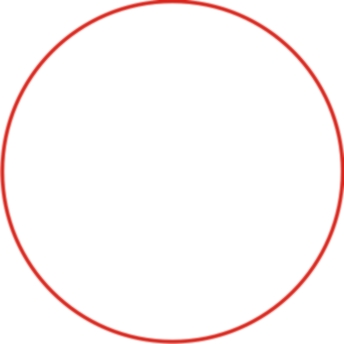A circle is a round plane figure whose distance from the circle to the edge is the same at any point.

Circle is a simple closed shape. It is the set of all points in a plane that are at a given distance from a given point, the centre.

A circle is a shape consisting of a curved line completely surrounding an area. Every part of the line is the same distance from the centre of the area.
So, do you now understand what a circle is?
Now let's take a practical example.
Examples of things circular in shapes (things that looks like a circle)
The wheel of a car
Coins
Plates
Sliced Oranges

## TERMS USED TO DESCRIBE A CIRCLE

Just like we have names, different complexions, different heights, which are used to identify us; Circles also have things used to identify them and differentiate them from other figures. In order to be able to solve questions under area of a circle, you must also be able to understand circle and it terms. The terms are listed below

### CIRCUMFERENCE OF A CIRCLE

This is the line surrounding a circular space, or the length of this line.
The outside edge of a circle or round object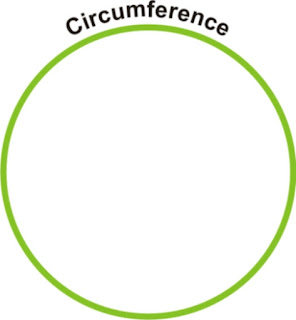The green line is the circumference of the circle. From that diagram above, a circumference could also be the path of a circle (basic definition )
Circumference of A Circle is also known as the perimeter of a circle.
Circumference = 2 * π * R
Along the line you find it in an image

### RADIUS OF A CIRCLE

This is the distance from the centre of the circle to any point on it edge
Radius is the distance from the centre of a circle to any point on the circumference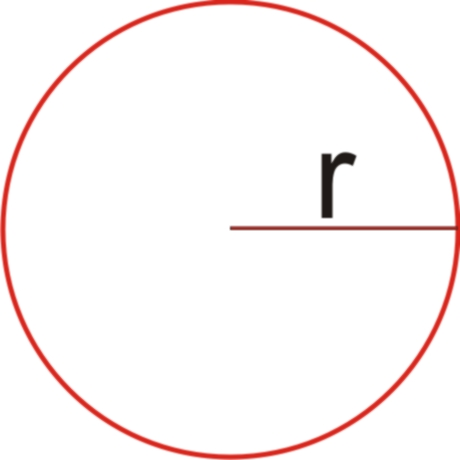The radius is the same at any point. It determines the size of the circle. A circle of radius 5cm is bigger than a circle of 2cm. Once you hear radius, the plane figure that comes to mind is circle.

### DIAMETER OF A CIRCLE

This is a straight line passing from one point of the circumference to the other side through the centre of a circle.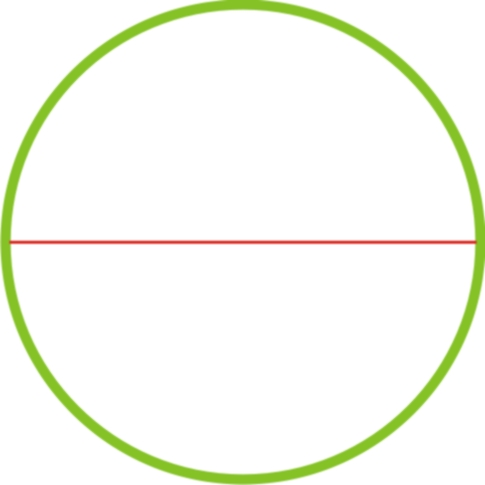The red line seen in the figure above is the diameter of the Circle. The diameter is the longest line touching two points on the circumference of the circle. It is denoted by  D.

## RELATIONSHIP BETWEEN DIAMETER AND RADIUS

In order to understand the relationship between a radius and a diameter, let�s use the images below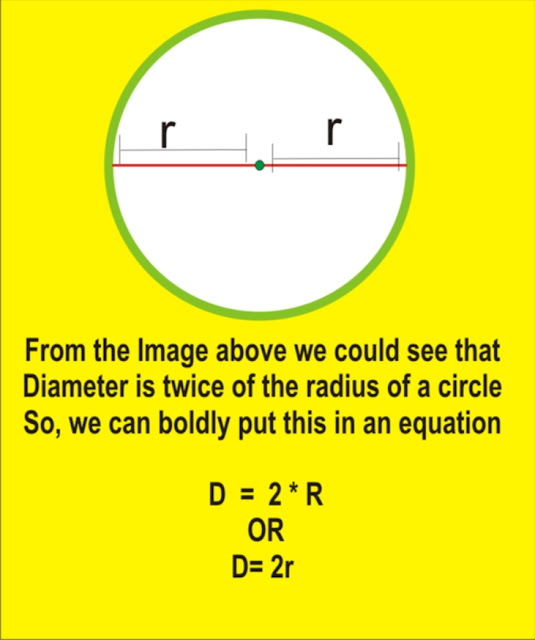Diameter = 2 X radius
AREA OF A CIRCLE

Definition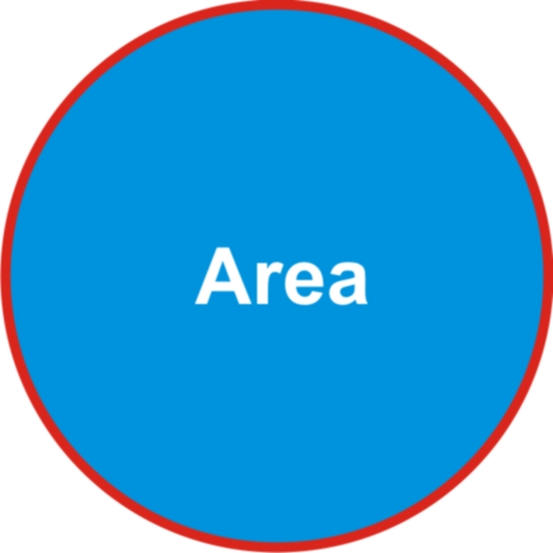The Blue part is the area of the circle.
Area of a circle is the total amount of a space in a circle

## How to Find the Area of a Circle Using Radius

AREA OF A CIRCLE=  π * SQUARE OF A THE CIRCLE RADIUS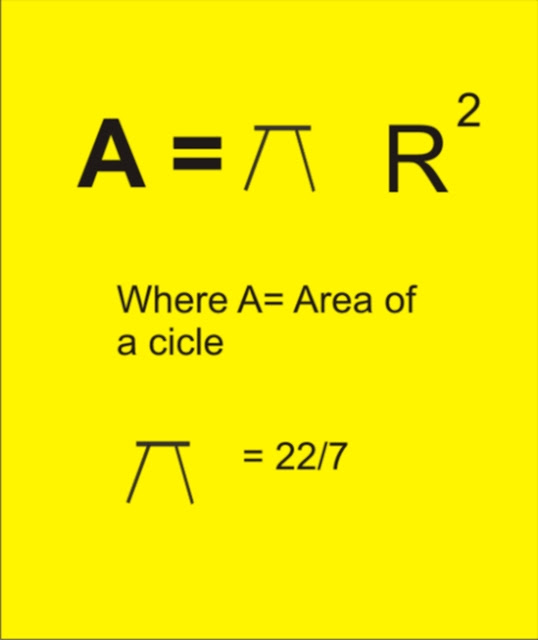## How to Find the Area of a Circle Using Diameter

In terms of diameter,  we have
Since we know that
D=2*R

Therefore,
R= D/2

Wherever you see radius, put D/2.

Area =π  *  (D/2)²

Perimeter of a Circle
This is the length of the circumference given as

Perimeter = 2 * π *R

In terms of diameter,
= π D
FOR EXAMPLE,
Calculate the area of a circle whose radius is 7cm.
Solution
From the formulae above, we know what to do.
π= 22/7